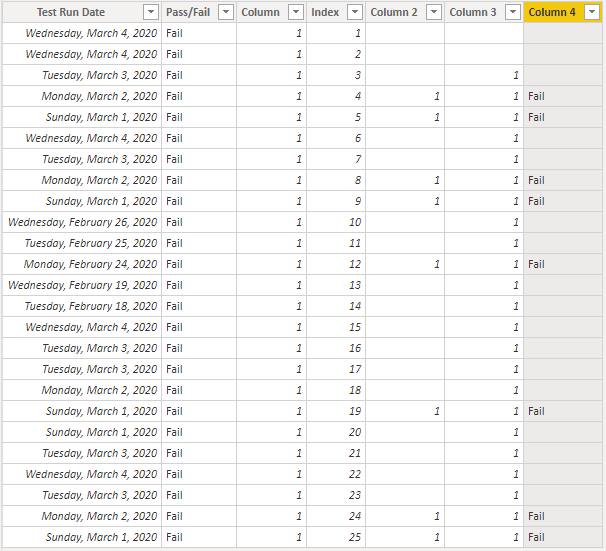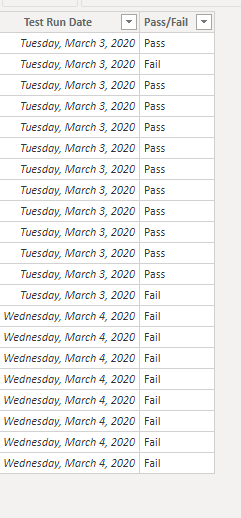cancel
Showing results for
Did you mean:

Fabric is Generally Available. Browse Fabric Presentations. Work towards your Fabric certification with the Cloud Skills Challenge.Anonymous
Not applicable

## Count Yes/No responses over 3 day time period. Return "Pass" if 3 consecutive 'Yes'. 'Fail' if not.

I have a table for test results with columns as follows:

Test Date / Metric / Source Value / Target Value / Difference / Pass/Fail

A test passes if the difference is 0. There are multiple tests over time for the same metric.

I want to count if a metric has 'Pass' in the Pass/Fail column consecutively every 3 days. Return a 'Pass' in a new column if so. 'Fail' if not.

I'm a Power BI newbie, any help would be appreciated!

1 ACCEPTED SOLUTIONCommunity Support

Hi @Anonymous ，

First go to query editor >add an index column,

Then create 4 columns as below:

``Column = IF(SEARCH("Fail",'Table'[Pass/Fail],,0)=0,0,1)``
``````Column 2 =
var pre1=CALCULATE(MAX('Table'[Test Run Date]),FILTER('Table','Table'[Index]=EARLIER('Table'[Index])-1))
var pre2=CALCULATE(MAX('Table'[Test Run Date]),FILTER('Table','Table'[Index]=EARLIER('Table'[Index])-2))
Return
``````Column 3 =
var pre1=CALCULATE(MAX('Table'[Pass/Fail]),FILTER('Table','Table'[Index]=EARLIER('Table'[Index])-1))
var pre2=CALCULATE(MAX('Table'[Pass/Fail]),FILTER('Table','Table'[Index]=EARLIER('Table'[Index])-2))
Return
IF(pre1=BLANK()||pre2=BLANK(),BLANK(),IF(pre1='Table'[Pass/Fail]&&pre2='Table'[Pass/Fail],1,BLANK()))``````
``Column 4 = IF('Table'[Column 2]=1&&'Table'[Column 3]=1,'Table'[Pass/Fail],BLANK())``

Finally you will see:Best Regards,
Kelly

7 REPLIES 7Super User

Can you share sample data and sample output.Anonymous
Not applicablefor your perusalAnonymous
Not applicable

Ignore Attribute columns.

As you can see, there can be multiple tests for the same metric on each day. I want to count if all those tests 'Pass' over 3 days.Community Support

Hi  @Anonymous ,

While analyzing your data,I found that there aren't 3 consecutive days in it,so would you pls check and update the data?Best Regards,
KellyAnonymous
Not applicable

See image. Ignore all 'Attribute' and 'UV?' columns.  There are around 2000 rows in this table.Community Support

Hi @Anonymous ，

First go to query editor >add an index column,

Then create 4 columns as below:

``Column = IF(SEARCH("Fail",'Table'[Pass/Fail],,0)=0,0,1)``
``````Column 2 =
var pre1=CALCULATE(MAX('Table'[Test Run Date]),FILTER('Table','Table'[Index]=EARLIER('Table'[Index])-1))
var pre2=CALCULATE(MAX('Table'[Test Run Date]),FILTER('Table','Table'[Index]=EARLIER('Table'[Index])-2))
Return
``````Column 3 =
var pre1=CALCULATE(MAX('Table'[Pass/Fail]),FILTER('Table','Table'[Index]=EARLIER('Table'[Index])-1))
var pre2=CALCULATE(MAX('Table'[Pass/Fail]),FILTER('Table','Table'[Index]=EARLIER('Table'[Index])-2))
Return
IF(pre1=BLANK()||pre2=BLANK(),BLANK(),IF(pre1='Table'[Pass/Fail]&&pre2='Table'[Pass/Fail],1,BLANK()))``````
``Column 4 = IF('Table'[Column 2]=1&&'Table'[Column 3]=1,'Table'[Pass/Fail],BLANK())``

Finally you will see:Best Regards,
KellySuper User

@Anonymous , where is the test completion date. I think not clear to me yet.Announcements#### Power BI Monthly Update - November 2023

Check out the November 2023 Power BI update to learn about new features.#### Fabric Community News unified experience

Read the latest Fabric Community announcements, including updates on Power BI, Synapse, Data Factory and Data Activator.#### The largest Power BI and Fabric virtual conference

130+ sessions, 130+ speakers, Product managers, MVPs, and experts. All about Power BI and Fabric. Attend online or watch the recordings.Top Solution Authors
Top Kudoed Authors
Users online (2,258)